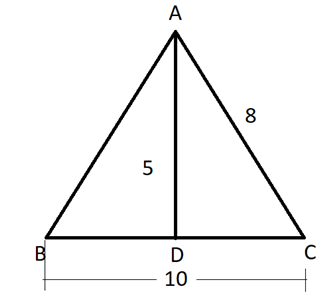We do not support landscape mode yet.
Please go back to portrait mode for the best experience
Question of The Day31-10-2019

A triangle ABC is made up of two sides AC and BC of dimension 8 cm and 10 cm respectively. A median of 5 m is drawn on the side BC. What is the dimension of the third side of the triangle?

Correct Answer : a ) 6

Explanation :

Drawing the schematic diagram of the triangle according to the given informationAs AD is the median so it will divide the line BC into two equal parts

Thus, BD = DC = 5 m

According to Apollonius theorem, “the sum of the squares of any two sides of any triangle is equal to twice the sum of square of the half of third side and square of the median bisecting the third side”

Therefore, applying Appolonius theorem in ? ABC

AB2 + AC2 = 2(AD2 + BD2)

So,

AB2 + 64 = 2 (25 + 25)

AB2 = 100 – 64

AB2 = 36

AB = 6 m

Hence, (a) is the correct answer.

Such type of question is asked in various government exams like SSC CGL, SSC MTS, SSC CPO, SSC CHSL, RRB JE, RRB NTPC, RRB GROUP D, RRB OFFICER SCALE-I, IBPS PO, RRB Office Assistant, IBPS Clerk,  RBIAssistant, IBPS RRB OFFICER SCALE 2&3, etc. Try and attempt free mock tests at PendulumEdu and learn easy techniques to solve such questions.